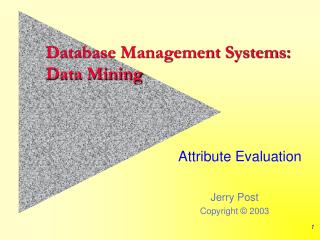DownloadDownload PresentationDatabase Management Systems: Data Mining

# Database Management Systems: Data Mining

Télécharger la présentation## Database Management Systems: Data Mining

- - - - - - - - - - - - - - - - - - - - - - - - - - - E N D - - - - - - - - - - - - - - - - - - - - - - - - - - -
##### Presentation Transcript

1. Database Management Systems:Data Mining Attribute Evaluation

2. Multiple Regression Y = b0 + b1X1 + b2X2 + … + bkXk Regression estimates the b coefficients. If a b value is zero, the corresponding X attribute does not influence the Y variable. The b value coefficient also indicates the strength of the relationship: dY/dXi = bi. A one unit increase in Xi results in a bi change in Y.

3. Regression Example: RT Query: Sales by Year by City Population: SELECT Format([orderdate],"yyyy") AS SaleYear, City.Population1990, Sum(Bicycle.SalePrice) AS SumOfSalePrice FROM City RIGHT JOIN (Customer INNER JOIN Bicycle ON Customer.CustomerID = Bicycle.CustomerID) ON City.CityID = Customer.CityID GROUP BY Format([orderdate],"yyyy"), City.Population1990 HAVING (((City.Population1990)>0)); Paste data into Exel. Tools/Data Analysis/Regression

4. Regression Results 75% variation explained Each year, sales increase \$356 Less than 0.05, so significantly different from zero For 1000 people, sales increase \$33

5. Information Gain: Partitioning In 1948, Shannon defined information (I) as: If pi is zero or one, there is no information—since you always know what will happen.

6. Information Example Types of shoppers (m=2): status is high roller or tourist S is a set of data (rows) The dataset contains attributes (A), such as: Income, Age_range, Region, and Gender. Each attribute has many (v) possible values. For example, Income categories are: low, medium, high, and wealthy. The subset Sij contains the rows of customers in category i who possess attribute level j. The count of the number of rows is sij. The entropy of attribute A defined from this partitioning is The information gain from the partitioning is Find the attribute with the highest gain.

7. Data for Information Example s1=104 s2=107 s=211 E(income)=0.2015 Gain(income) = 0.9999-0.2015 = 0.7984 =79/211*I(…)

8. Results for Information All values are relatively high, so all attributes are important.

9. Dimensionality • Notice the issue of dimensionality in the example. • We had to setup groups within the attributes. • If there are too many groupings/values: • The system will take a long time to run. • Many subgroups will have no observations. • How do you establish the groupings/values? • Natural hierarchies (e.g., dates) • Cluster analysis • Prior knowledge • Level of detail required for analysis

10. Non-Linear Estimation • Regression: • Polynomial: Y = b0 + b1X + b2X2 + b3X3 + b4X4…+ u • Exponential: Y = b0Xb1eu ln(Y) = ln(b0) + b1 ln(X) + u • Log-Linear: ln(Y) = b0 + b1 ln(X) + u • Other: log log and more • Other Methods: • Neural networks • Search

11. Example: PolyAnalyst: Find Law for MPG mpg = (2.59183e+009 *power*age+176465 *power*age*weight+2.41554e+009 *power*age*age-3.54349e+009 *power+7.27281e+007 *age*weight-2.55635e+010)/(power*age*weight+52028.3 *power*age*age*weight) Best exact rule found: mpg = (4.71047e+008 *power*age*weight-38783.5 *power*age*weight*weight+2.5987e+009 *power*age*age*weight-7.65205e+009 *power*weight+1.5658e+008 *age*weight*weight+1.15859e+011 *power*power-3.0532e+013 *age*age)/(power*age*weight*weight+52028.3 *power*age*age*weight*weight)

12. MPG Versus Weight

13. Problems with Non-Linear Models • They can be harder to estimate. • They are substantially more difficult to optimize. • They are often unstable—particularly at the ends. Y = 15000 – 850 X – 435 X2 + 2 X3 + X4 Note: (x + 7)(x – 5)(x + 20)(x – 20)